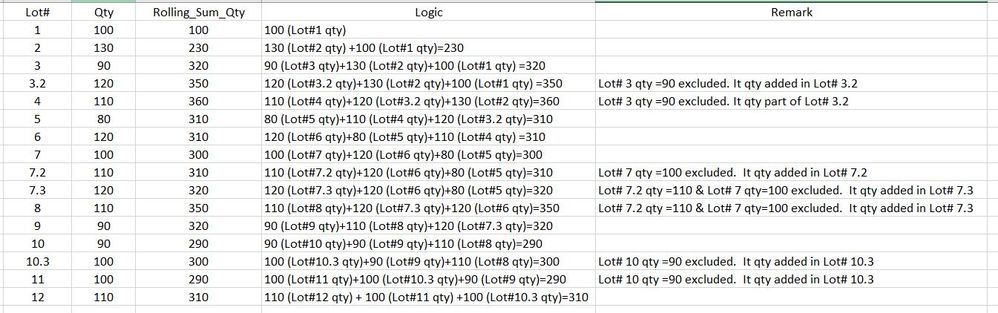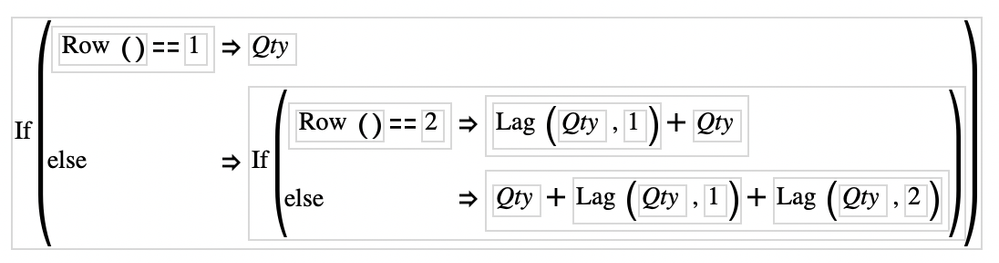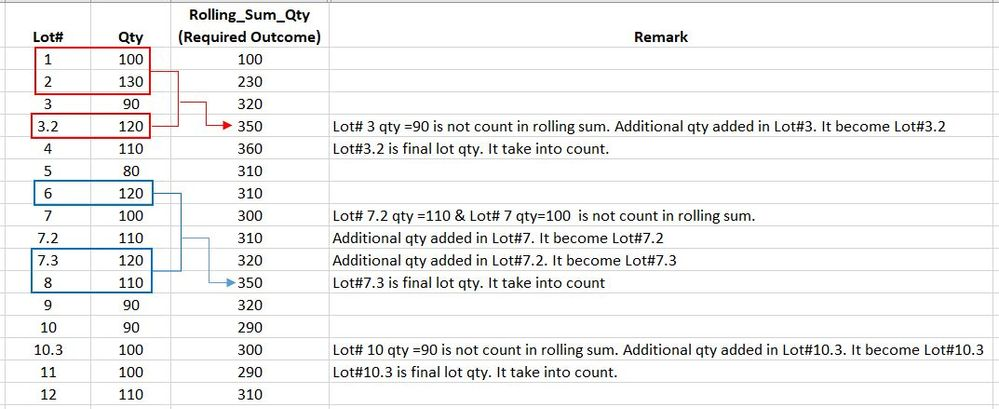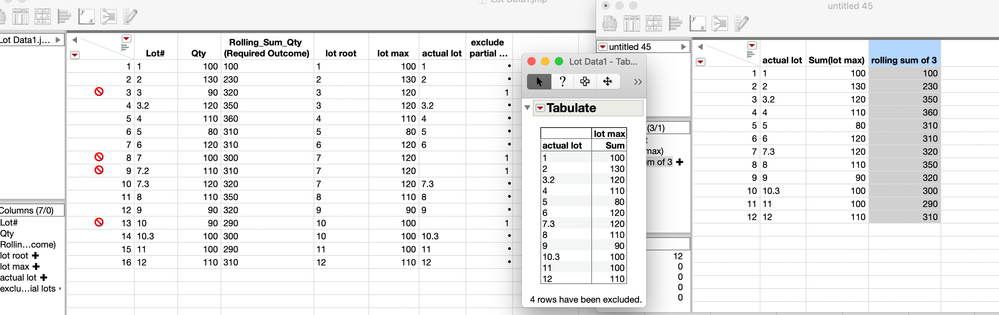Our World Statistics Day conversations have been a great reminder of how much statistics can inform our lives. Do you have an example of how statistics has made a difference in your life? Share your story with the Community!
Choose Language Hide Translation Bar
Highlighted

## Rolling Sum of Previous Row Values with Condition

My data starts with two column, Lot# & Qty.

I need help to create script to calculate rolling sum (current row + previous 2 rows) of previous row values.

The logic is explained as below table.1 ACCEPTED SOLUTION

Accepted Solutions
Highlighted

## Re: Rolling Sum of Previous Row Values with Condition

Add this script to you data table and give it a spin

``````dt1=current data table();
//I want to get the max quantity by lot, so I'm adding a new column to change all the lots back to their non-decimal value.
dt1<< New Column("Lot Actual", formula(If( :Qty == Col Maximum( :Qty, Char( Round( Num( :Lot# ), 0 ) ) ),
:Lot#,
.
)));//this next formula column excludes rows that aren't the max Qty by lot
dt1<< New Column( "Lot Values in One Formula",
Formula(
If( :Lot Actual == "",
Row State( row() ) = Excluded State( 1 );
Excluded( Row State( row() ) );
1;
)
)
);
dt1<<run formulas();
//once all the formulas for tagging the data are added, we can use Tabulate to get a subset of just the important rows.//There are lots of other ways to do this, this just happened to be the method of the day

obj1=dt1 << Tabulate(
Column Table( Analysis Columns( :Qty ) ),
Row Table( Grouping Columns( :Lot# ) )
)
);
dt2=obj1 << Make Into Data Table;
column(dt2,2)<<set name("Lot Max");//Finally here is the rolling sum of the last three lots//there is moving average function we could have used, it would have looked simpler but more abstract
dt2<<new column("Rolling Sum of 3",formula(If( Row() == 1,
:Name( "Lot Max" ),
If( Row() == 2,
Lag( :Name( "Lot Max" ), 1 ) + :Name( "Lot Max" ),
:Name( "Lot Max" ) + Lag( :Name( "Lot Max" ), 1 )
+Lag( :Name( "Lot Max" ), 2 )
)
)));
////This part joins the values from the separate table back to the original table
dt1 << Update(
With( dt2 ),
Match Columns( :Lot# = :Lot# )
);
close(dt2, nosave);
obj1<<close window;
dt1<< Delete Columns("Lot Actual", "Lot Max", "Lot Values in One Formula");

/////I feel like this step is cheating a little. This step just repeats the missing values down to the next non-missing//row. Its some code I got from Brady Brady a ways back. Different iterations of come in handy from time to time.
dtnr=current data table();

dtcollist=dtnr<<Get Column Names;
dtMat = (dtnr << Get All Columns As Matrix);
selection={}; insert into (selection, column(4)); //these columns get acted on
For( i = 1, i <= N Items( selection ), i++,
colNum = Contains( dtColList, Name Expr( selection[i] ) );
vMat = dtMat[0, colNum];
mis = Loc( Is Missing( vMat ) );
If( N Row( mis ),
col = selection[i];
If( mis == 1,
col = col[(Loc( vMat ))]
);

For( j = if(mis==1, 2, 1), j <= N Row( mis ), j++,
col[mis[j]] = col[mis[j] - 1]
);
);
);``````
JMP Systems Engineer, Pharm and BioPharm Sciences
9 REPLIES 9
Highlighted

## Re: Rolling Sum of Previous Row Values with Condition

One way might be to use a formula like this:``````If( Row() == 1,
:Qty,
If( Row() == 2,
Lag( :Qty, 1 ) + :Qty,
:Qty + Lag( :Qty, 1 ) + Lag( :Qty, 2 )
)
)``````

For the first row there is nothing to sum, so it just uses row 1

For the second row there are only two values, the current and previous, so its row+lag(1)

All rows after the second it takes the sum of the current row plus the previous two rows :lag(1) and lag(2)

JMP Systems Engineer, Pharm and BioPharm Sciences
Highlighted

## Re: Rolling Sum of Previous Row Values with Condition

Thanks for proving idea of using formula.

My dataset is not able to do rolling sum directly using the formula because some lot data is not take into count.

Example,

Lot# 3 and Lot# 3.2, only Lot# 3.2 qty is count in rolling sum because it is final lot qty.

It is the same for Lot# 7, 7,2, 7,3 and Lot# 10, 10.3.

Lot# 7.3 qty and 10.3 is count in rolling sum because it is final lot qty.

Need guidance from the community.Highlighted

## Re: Rolling Sum of Previous Row Values with Condition

Right, I get it now.

Need to find the max value for each lot, then take the rolling sum of the current and last two max lot values.

I feel like there is a lurking solution, might need to think a little more on that one.

JMP Systems Engineer, Pharm and BioPharm Sciences
Highlighted

## Re: Rolling Sum of Previous Row Values with Condition

I will figure out how to get this.

Just in case anyone has solution for this, please share as well.

Thanks.

Highlighted

## Re: Rolling Sum of Previous Row Values with Condition

Is this right. (the right most column in the picture)?JMP Systems Engineer, Pharm and BioPharm Sciences
Highlighted

## Re: Rolling Sum of Previous Row Values with Condition

Yes, that is right.

But I want to maintain the original data table without excluding some rows to get this result.

Can this be done?

Highlighted

## Re: Rolling Sum of Previous Row Values with Condition

Add this script to you data table and give it a spin

``````dt1=current data table();
//I want to get the max quantity by lot, so I'm adding a new column to change all the lots back to their non-decimal value.
dt1<< New Column("Lot Actual", formula(If( :Qty == Col Maximum( :Qty, Char( Round( Num( :Lot# ), 0 ) ) ),
:Lot#,
.
)));//this next formula column excludes rows that aren't the max Qty by lot
dt1<< New Column( "Lot Values in One Formula",
Formula(
If( :Lot Actual == "",
Row State( row() ) = Excluded State( 1 );
Excluded( Row State( row() ) );
1;
)
)
);
dt1<<run formulas();
//once all the formulas for tagging the data are added, we can use Tabulate to get a subset of just the important rows.//There are lots of other ways to do this, this just happened to be the method of the day

obj1=dt1 << Tabulate(
Column Table( Analysis Columns( :Qty ) ),
Row Table( Grouping Columns( :Lot# ) )
)
);
dt2=obj1 << Make Into Data Table;
column(dt2,2)<<set name("Lot Max");//Finally here is the rolling sum of the last three lots//there is moving average function we could have used, it would have looked simpler but more abstract
dt2<<new column("Rolling Sum of 3",formula(If( Row() == 1,
:Name( "Lot Max" ),
If( Row() == 2,
Lag( :Name( "Lot Max" ), 1 ) + :Name( "Lot Max" ),
:Name( "Lot Max" ) + Lag( :Name( "Lot Max" ), 1 )
+Lag( :Name( "Lot Max" ), 2 )
)
)));
////This part joins the values from the separate table back to the original table
dt1 << Update(
With( dt2 ),
Match Columns( :Lot# = :Lot# )
);
close(dt2, nosave);
obj1<<close window;
dt1<< Delete Columns("Lot Actual", "Lot Max", "Lot Values in One Formula");

/////I feel like this step is cheating a little. This step just repeats the missing values down to the next non-missing//row. Its some code I got from Brady Brady a ways back. Different iterations of come in handy from time to time.
dtnr=current data table();

dtcollist=dtnr<<Get Column Names;
dtMat = (dtnr << Get All Columns As Matrix);
selection={}; insert into (selection, column(4)); //these columns get acted on
For( i = 1, i <= N Items( selection ), i++,
colNum = Contains( dtColList, Name Expr( selection[i] ) );
vMat = dtMat[0, colNum];
mis = Loc( Is Missing( vMat ) );
If( N Row( mis ),
col = selection[i];
If( mis == 1,
col = col[(Loc( vMat ))]
);

For( j = if(mis==1, 2, 1), j <= N Row( mis ), j++,
col[mis[j]] = col[mis[j] - 1]
);
);
);``````
JMP Systems Engineer, Pharm and BioPharm Sciences
Highlighted

Thanks Byron.

Highlighted

## Re: Rolling Sum of Previous Row Values with Condition

Thanks for posting your question. That was a fun, but twisty problem to work out.

I added some annotation to the JSL, hopefully is makes sense and doesn't just look like a giant magic formula.

Honestly, at first I thought it would be nearly impossible to sort out, but after bigger cup of coffee,.. not so bad.

JMP Systems Engineer, Pharm and BioPharm Sciences
Article Labels